# SAT Math: Exponents Chapter Exam

Exam Instructions:

Choose your answers to the questions and click 'Next' to see the next set of questions. You can skip questions if you would like and come back to them later with the yellow "Go To First Skipped Question" button. When you have completed the practice exam, a green submit button will appear. Click it to see your results. Good luck!

### Page 1

#### Question 1 1. Which of the following is equivalent to the expression below?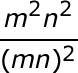#### Question 2 2. Simplify the expression using positive exponents.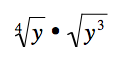#### Question 3 3. Which of the following exponential expressions is equal to this radical expression?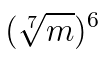#### Question 4 4. In the equation shown, what is the value of b?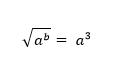#### Question 5 5. Which of the following would be true regarding the following exponential expression?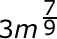### Page 2

#### Question 6 6. Simplify the following expression.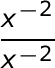#### Question 7 7. Evaluate: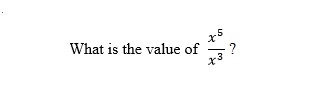#### Question 8 8. Evaluate: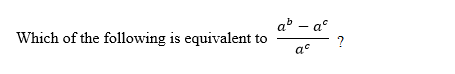#### Question 9 9. Using positive exponents, simplify the expression.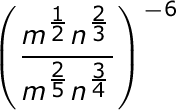#### Question 10 10. Simplify the following expression using positive exponents.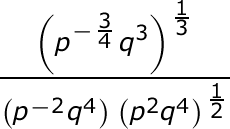### Page 3

#### Question 11 11. Which of the following is equivalent to the expression below?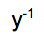#### Question 12 12. Simplify the expression below using only positive exponents.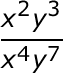#### Question 13 13. Simplify the following expression.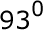#### Question 14 14. Evaluate: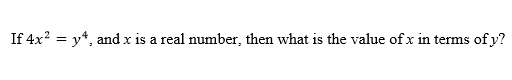#### Question 15 15. Evaluate: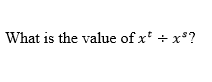### Page 4

#### Question 16 16. Simplify using ONLY positive exponents.#### Question 17 17. Simplify this expression using positive exponents.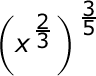#### Question 18 18. Which of the following is equivalent to the expression shown: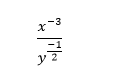#### Question 19 19. Simplify the expression below.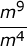#### Question 20 20. Evaluate: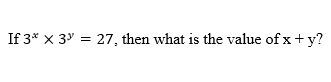### Page 5

#### Question 21 21. Simplify the following expression using positive exponents only.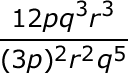#### Question 22 22. Evaluate: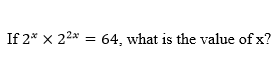#### Question 23 23. Which of the following is equivalent to the expression below?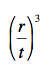#### Question 24 24. In the equation given, what is the value of y?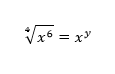#### Question 25 25. Which of the following is equivalent to the following expression?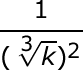### Page 6

#### Question 26 26. Which of the following is equivalent to the expression below?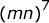#### Question 27 27. Which of the following radical expressions is equal to this exponential expression?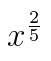#### Question 28 28. Evaluate: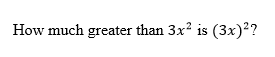#### Question 29 29. Simplify the expression below using positive exponents.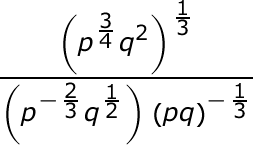#### Question 30 30. Which of the following is equivalent to the following expression?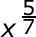#### SAT Math: Exponents Chapter Exam Instructions

Choose your answers to the questions and click 'Next' to see the next set of questions. You can skip questions if you would like and come back to them later with the yellow "Go To First Skipped Question" button. When you have completed the practice exam, a green submit button will appear. Click it to see your results. Good luck!

Support